# DQM QUESTION

Hi all, I would like to ask how the equation is implemented problem? https://github.com/dwave-examples/airline-hubs/blob/main/demo.py

Can anybody decode or give step by step explanation(build_dqm function) on how the equation is converted to dqm?

1 comment
• Hi Ijas,

Apologizes for the delayed response! It took us some time to get back to you.

The formulation used in this example is based on the paper: O'Kelly, Morton E. "A quadratic integer program for the location of interacting hub facilities."

Problem: In the demo, we have a list of 25 airports(city-data.txt) and for each inter-city travel or leg we have a cost(cost.csv) and passenger demand(flow.csv) available. The problem is to find 3 hub locations, which can act as switching points between airports, while minimizing the cost of operation.

Problem Formulation: For each airport, either it can connect to a hub or it can be a hub, which gives each airport 25 discrete values to choose from. Consider ATL airport as a variable(X_ATL), which represent the airport it directly connects with, if X_ATL = SFO that means you can fly direct from ATL to SFO or if X_ATL = ATL then ATL is a hub.

In `dimod.DiscreteQuadraticModel`, we represent each airport as a variable and the discrete values it can take as cases. It can be denoted as X_{i,j}, where i is a variable and j is a case. It can be thought of as a binary variable: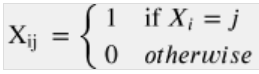So, for the above example, if ATL is directly connected to SFO then X_{ATL,SFO}=1 and rest 24 cases will be zero.

In the build_dqm function, a DQM object is created and for each airport, a variable is created and each variable gets 25 cases(one for each city).

The code implements the objective function that's outlined in the paper, linear terms evaluate the cost of assigning a node to its hub and the quadratic term evaluates the cost of interaction between the hubs. Here C_{ij} is the cost of operating a direct route from city i to city j and W_{ij} is the passenger demand from city i to city j.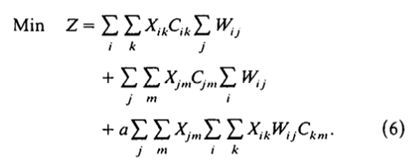Constraint1: Every leg must connect to a hub

One hot implementation of the DQM solver ensures that each airport gets assigned to one and only one hub and the first constraint enforces that an airport only connects to a hub

So, if X_{ij}= 1 then this constraint ensures X_{jj}= 1.

The table of all outcomes: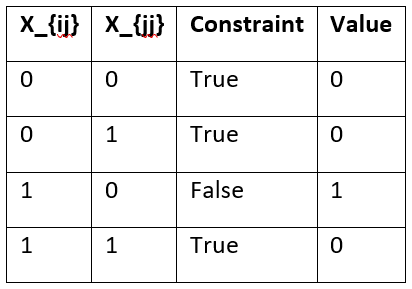Working through the truth table, we get the following equation that satisfies the above:Constraint2: Exactly p hubs required

Sum of the X_{jj} should be p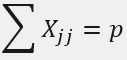which can be simplified to(refer section 4.3 in our problem formulation guide for details),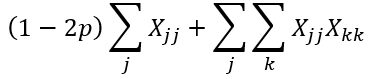Each summation in the above equations is implemented by a for loop in the code. The full DQM is constructed by adding the objective function and the weighted constraints(using Lagrange parameter, gamma1 and gamma2).

Also, if you would like to learn more about DQM, we have an upcoming webinar on May 25 which will cover problem formulation using DQM and this example in detail. You can register for it here or watch the recording on our youtube channel after the webinar.

I hope this helps! Please let us know if you have any questions.